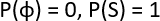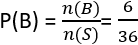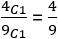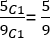You are here
Home > Class 11 > Probability Class 11

# Probability Class 11Chapter 16

ProbabilityIn earlier class, we have already studied about the concept of probability. We have defined the probability of an event as the ratio of number of outcomes favorable to the event, to the total number of outcomes.

Random experiments

In our day today life, there are so many experimental activities, the results may not be same when they are repeated under same conditions. Consider the following experiments

Tossing a coin

Throwing a die

We know the results of these experiments. But we are not sure which one of these results will come when it is executed. These kinds of experiments are called random experiments

The results of a random experiments are called outcomes

The set of all possible outcomes of a random experiment is called sample space and is denoted by s

Write the sample space of the following random experiments

1 Tossing a coin

S = {H,T}

2. Tossing 2 coins

S = {HH,HT,TH,TT}

3. Tossing 3 coins

S = {HHH, HTT,THT,TTH,THH,HTH,HHT,TTT}

2n is the number of elements in the sample space of tossing n coins

4. Throwing a die

S= {1,2,3,4,5,6}

5. Throwing 2 dice

S = {(1,1)…(6,6)}

6n is the number of elements in the sample space of throwing n dice

6. A coin is tossed. If it shows a tail, we draw a ball from a box containing 2 red and 3 black balls. If it shows head, we throw a die

Le R1,R2 be the red balls and B1,B2,B3 be the black balls

S = {TR1,TR2,TB1,TB2,TB3,H1,H2,H3,H4,H5,H6}

7.  A coin is tossed until a head comes up

S = {H,TH,TTH,TTTH,…}

Event

The subset of a sample space is called event

S = {1,2,3,4,5,6}

A = {2,4,6}

B= {1,3,5}

C= {2,3,5}

S is called sure event and ⌽   is called impossible event

Mutually exclusive events

If A B = , then A and B are called mutually exclusive events

S = {1,2,3,4,5,6}

A = {2,4,6}

B= {1,3,5}

A ⋂  B = ⌽

A and B are mutually exclusive

Exhaustive events

Two or more events are exhaustive if their union is the sample space

S = {1,2,3,4,5,6}

A = {1,2,3}

B = {3,5}

C = {4,5,6}

A ⋃ B ⋃ C = S

A = {1,2,3}

C = {4,5,6}

Probability of an event

Probability of the event a is denoted by P(A) and is defined asQ. A die is thrown. Find the probability of the following events

(i) getting an even number

S = {1,2,3,4,5,6}

A = {2,4,6}(II) getting an odd number

B = {1,3,5}(iii) Getting a prime number

C = {2,3,5}Q. 2 dice are thrown. Find the probability of the following events

(i) getting a sum of 8

S = {(1,1),…,(6,6)}

A = {(5,3),(3,5), (6,2),(2,6),(4,4)}(ii) getting a doublet

B = {(1,1),(2,2),(3,3),(4,4),(5,5),(6,6)}(iii)at least one of the dice shows 4

C= {(1,4),(4,1),(2,4),(4,2),(3,4),(4,3),(4,4),(5,4),(4,5),(6,4),(4,6)}Q. A bag contains 4 red
and 5 black balls. A ball is drawn at random. Find the probability that

(i)the ball is red(ii) The ball is blackStay tuned

The page is under construction.